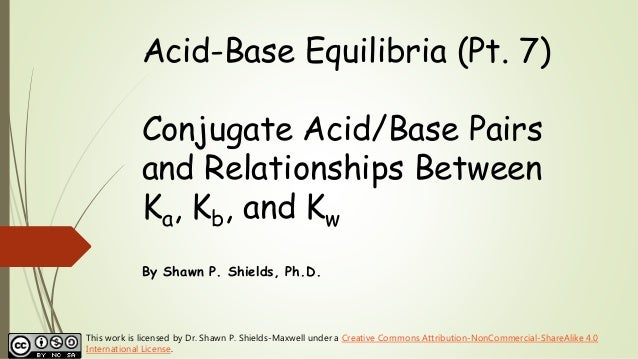Successfully reported this slideshow.
We use your LinkedIn profile and activity data to personalize ads and to show you more relevant ads. You can change your ad preferences anytime.Upcoming SlideShare
Loading in …5
×

# Chem 2 - Acid-Base Equilibria VII: Conjugate Acid/Base Pairs and Relationships Between Ka, Kb, and Kw

Chem 2 - Acid-Base Equilibria VII: Conjugate Acid/Base Pairs and Relationships Between Ka, Kb, and Kw

• Full Name
Comment goes here.

Are you sure you want to Yes No
Your message goes here• Login to see the comments

### Chem 2 - Acid-Base Equilibria VII: Conjugate Acid/Base Pairs and Relationships Between Ka, Kb, and Kw

1. 1. Acid-Base Equilibria (Pt. 7) Conjugate Acid/Base Pairs and Relationships Between Ka, Kb, and Kw By Shawn P. Shields, Ph.D. This work is licensed by Dr. Shawn P. Shields-Maxwell under a Creative Commons Attribution-NonCommercial-ShareAlike 4.0 International License.
2. 2. Recall: Conjugate Acid-Base Pairs Conjugate acid-base pairs are two related species differing only by a proton (H+). 𝐇𝐅 𝐚𝐪 + 𝐇 𝟐 𝐎 𝐥 ⇌ 𝐇 𝟑 𝐎+ 𝐚𝐪 + 𝐅− (𝐚𝐪) 𝐍𝐇 𝟑 𝐚𝐪 + 𝐇 𝟐 𝐎 𝐥 ⇌ 𝐍𝐇 𝟒 + 𝐚𝐪 + 𝐎𝐇− (𝐚𝐪) base conjugate acid for NH3 acid conjugate base for HF
3. 3. The equilibrium constant K is “renamed” for acids to Ka The Equilibrium Constant Ka for Weak Acids An equilibrium exists between the weak acid (HA) and its products. 𝐇𝐀 𝐚𝐪 + 𝐇 𝟐 𝐎 𝐥 ⇌ 𝐇 𝟑 𝐎+ 𝐚𝐪 + 𝐀− (𝐚𝐪) 𝐊 𝐚 = 𝐇 𝟑 𝐎+ 𝐀− 𝐇𝐀
4. 4. Ka and Kb for Conjugate Acid-Base Pairs Suppose acetic acid (CH3COOH) is dissolved in water… 𝐂𝐇 𝟑 𝐂𝐎𝐎𝐇 𝐚𝐪 + 𝐇 𝟐 𝐎 𝐥 ⇌ 𝐂𝐇 𝟑 𝐂𝐎𝐎− 𝐚𝐪 + 𝐇 𝟑 𝐎+ 𝐚𝐪 acetic acid conjugate base for acetic acid “loseable” H+
5. 5. Ka and Kb for Conjugate Acid-Base Pairs Suppose acetate (CH3COO-) is dissolved in water… 𝐂𝐇 𝟑 𝐂𝐎𝐎− + 𝐇 𝟐 𝐎 𝐥 ⇌ 𝐂𝐇 𝟑 𝐂𝐎𝐎𝐇 𝐚𝐪 + 𝐎𝐇− (𝐚𝐪) acetate conjugate acid for acetate acetate gained H+ from water
6. 6. Ka and Kb for Conjugate Acid-Base Pairs CH3COOH and CH3COO- are conjugate acid-base pairs acetic acid acetate (base) Ka = 1.76  10-5 Kb = 5.68  10-10
7. 7. Multiply Ka and Kb for Conjugate Acid-Base Pairs 𝐊 𝐚 ∙ 𝐊 𝐛= 𝟏. 𝟕𝟔 × 𝟏𝟎−𝟓 [𝟓. 𝟔𝟖 × 𝟏𝟎−𝟏𝟎 ] 𝐊 𝐚 ∙ 𝐊 𝐛= 𝟏 × 𝟏𝟎−𝟏𝟒 = 𝐊 𝐖 𝐊 𝐚 ∙ 𝐊 𝐛 = 𝐊 𝐖 Use this relationship to interconvert between Ka and Kb.
8. 8. Calculating (Converting Between) Ka and Kb using Kw 𝐊 𝐚 ∙ 𝐊 𝐛 = 𝐊 𝐖 𝐊 𝐚 ∙ 𝐊 𝐛= 𝟏. 𝟎 × 𝟏𝟎−𝟏𝟒 = 𝐊 𝐖 𝐊 𝐚 = 𝐊 𝐖 𝐊 𝐛 𝐊 𝐛 = 𝐊 𝐖 𝐊 𝐚 Use KW to interconvert between Ka and Kb.
9. 9. Calculating (Converting Between) Ka and Kb using Kw 𝐊 𝐚 ∙ 𝐊 𝐛= 𝟏. 𝟕𝟔 × 𝟏𝟎−𝟓 [𝟓. 𝟔𝟖 × 𝟏𝟎−𝟏𝟎 ] 𝐊 𝐚 ∙ 𝐊 𝐛= 𝟏. 𝟎 × 𝟏𝟎−𝟏𝟒 = 𝐊 𝐖 𝐊 𝐚 = 𝟏.𝟎×𝟏𝟎−𝟏𝟒 𝟓.𝟔𝟖×𝟏𝟎−𝟏𝟎 𝐊 𝐛 = 𝟏.𝟎×𝟏𝟎−𝟏𝟒 𝟏.𝟕𝟔×𝟏𝟎−𝟓 Use KW to interconvert between Ka and Kb.
10. 10. pKa and pKb Calculate the pKa for an acid as pKa =  log Ka Calculate the pKb for a base as pKb =  log Kb
11. 11. Calculating pKa and pKb Calculate the pKa pKa =  log Ka 𝐩𝐊 𝐚 = −𝐥𝐨𝐠 𝟏. 𝟕𝟔 × 𝟏𝟎−𝟓 = 𝟒. 𝟕𝟓 Calculate the pKb pKb =  log Kb 𝐩𝐊 𝐛 = −𝐥𝐨𝐠 𝟓. 𝟔𝟖 × 𝟏𝟎−𝟏𝟎 = 𝟗. 𝟐𝟓
12. 12. Inverse logs for pKa, pKa, and pKw pKa =  log Ka 10pKa = Ka pKb =  log Kb 10pKb = Kb pKw =  log Kw = 14 10pKw = Kw Recall: Kw (and pKw) will have different values at temperatures other than 25C
13. 13. A Few More Relationships Between pKa, pKb, and pKw pKa + pKb = pKw pKw =  log [Kw] = 14 (at 25C) pKa + pKb = 14 (at 25C)
14. 14. Next up, The Conjugate See-Saw and Analyzing Ka and Kb for Acid or Base Relative Strength (Pt. 8)